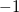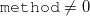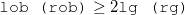Q-Chem 4.3 User’s Manual

# 10.20 Quantum Transport Properties via the Landauer Approximation

Quantum transport at the molecule level involves bridging two electrodes with a molecule or molecular system, and calculating the properties of the resulting molecular electronic device, including current-voltage curves, the effect of the electrodes on the molecular states, etc. For a general introduction to the field, the following references are useful [578, 579].

The quantum transport code in Q-Chem is developed by Prof. Barry Dunietz (Kent State) and his group. This package is invoked by the \$rem variable TRANS_ENABLE.

TRANS_ENABLE
 Decide whether or not to enable the molecular transport code.

TYPE:
 INTEGER

DEFAULT:
 0 Do not perform transport calculations.

OPTIONS:
 1 Perform transport calculations in the Landauer approximation.Print matrices for subsequent calls for tranchem.exe as a stand-alone post-processing utility, or for generating bulk model files.

RECOMMENDATION:
 Use as required.

Output is provided in the Q-Chem output file and in the following additional files:

• transmission.txt (transmission function in the requested energy window)

• TDOS.txt

• current.txt (I-V plot if set)

• FAmat.dat (Hamiltonian matrix for follow up calculations and analysis)

• Smat.dat (Hamiltonian matrix for follow up calculations and analysis)

T-Chem requires two parameter files:

• Trans-model.para (Specifies the cluster model of the molecular device)

• Trans-method.para (Specifies the method(s) used in the transport calculation)

All the numbers must be provided. The details of file formats are given in Table 10.1 and Table 10.2.

 Input example Explanation 142 Totorb total number of atomic orbitals in the cluster model of the molecular junction 22 lob number of AO’s representing the left electrode (the first lob functions of input) 22 rob number of AO’s representing the right electrode (the last rob functions of input) 22 lg size of the repeating unit of the left electrode 22 rg size of the repeating unit of the right electrode 22 ql must be set to the lg value (later used in NEGF optimizations) 22 qr must be set to the rg value (later used in NEGF optimizations)
Table 10.1: Example of a Trans-model.para file, and the meaning of each input line. All lines must be present

Key points to note about the Trans-model.para file, as documented in Table 10.1:

• WARNING: Unphysical setting of the regions is not caught by the program and might (will!) produce unphysical transmission functions. For example, the cluster model can be accidently partitioned by mistake within the orbital space of an atom. In fact, of course, It should always be partitioned between atomic layers.

• The ordering of the atoms in the \$molecule section of the Q-Chem input file IS important and is assumed to be as follows: Repeating units (left) - Molecular Junction - Repeating units (right).

• The atoms are provided by sets of left electrode repeating units first, in order of most distant from the surface layer. Then comes the the molecular region, followed by the right electrode region which starts with the surface layer to end with the most distant layer. The atom order within each layer (each repeating unit) must be consistent.

 Input example Keywords included on each line 1 enable 0 AB -6.5 -8.5 -4.5 300 efermi-emin-emax-npoints 0 0 0.01 0.01 0.07 method-htype-device-bulkr_smear-green_const 0 1 1 readinHS-tot-start 4.0 4 100 1.0 vmax-numres-numlinear-lpart 1 2 100 printDOS-printIV-ipoints 298.15 IVtemp
Table 10.2: Example of a Trans-method.para file, and the keywords that correspond to each input line. The supported values of each keyword is listed below. All lines must be present.

The allowed values of the various keywords that comprise each line of the Trans-method.para file follow.

• enable: Sets the type of calculation. Allowed values are:

• 1: Landauer level

• 3: SCF GF at V=0 (not yet enabled)

• 4: Full NEGF (not yet enabled)

• AB: Determines spins. Only closed shell singlet (0) allowed at present

• emin,emax,npoints: Energy window for which transmission is calculated (eV), and number of points

• method: Scheme to calculate the electrode GFs:

• 0: A wide band limit (WBL) with a constant parameter (greens_const)

• 1: WBL following the procedure proposed by Ke-Baranger-Yang

• 2: WBL following the procedure proposed by Lopez-Sancho (decimation). Recommended

• 3: Full TB following the procedure proposed by Ke-Baranger-Yang

• 4: Full TB following the procedure proposed by Lopez-Sancho (decimation). Recommended

• Comment: At least a single unit of the repeating layer of the electrodes is required to be included in the junction region. Another layer has to be included if readinhs==0 and a total of two additional layers are needed if.

• htype: determines the electronic coupling terms used for the self energy calculations:

• 0: all coupling integrals between the junction and electrode are set following the cluster model (no screening imposed)

• 1: only coupling between neighboring repeating units of the electrode model is allowed

• 2: set the coupling terms by the electrode models that are read in (readinhs=1) or by the sufficiently large cluster model, where• device-smear: Imaginary smearing (eV) added to the real Hamiltonian for device GF evaluation.

• bulk-smear: Imaginary smearing (eV) added to the real Hamiltonian for electrodes GF evaluation.

• 0: no electrode Hamiltonians are available

• 1: pre-calculated electrode Hamiltonians will be used. Expected files are FAmat2l.dat, FBmat2l.dat, FAmat2r.dat, FBmat2r.dat, Smat2l.dat, Smat2r.dat. totorb2 is the total number of basis functions in the electrode model (same size is assumed for both electrodes), and, start is the first basis function from which the TB integral are to be extracted from.

• vmax, numres, numlinear, lpart

• vmax = voltage should be equal to emax-emin if IV is set

• other parameters here define the integration path for the NEGF algorithm (not used in this version, place holders must be included)

• printDOS

• 0: no total dos printing

• 1: a TDOS (of the junction region) will be printed as TDOS.txt

• printIV (and ipoints, IVtemp)

• 0: no I-V information is calculated

• 1: I(V=Vmax) is printed in current.txt

• 2: I-V for bias from 0 to Vmax with a grid of ipoints at IVtemp is evaluated and printed in current.txt

As an example, the sample Q-Chem input that works with the files documented in Table 10.1 and Table 10.2 is given below.

Example 10.238  Quantum transport calculation applied to Cbetween two gold electrodes.

```\$molecule
0 1
Au -0.2 0 0
Au  2.5 0 0
C   4.8 0 0
C   6.5 0 0
C   8.2 0 0
C   9.9 0 0
C  11.6 0 0
C  13.3 0 0
Au 15.6 0 0
Au 18.3 0 0
\$end

\$rem
jobtype               SP
method                B3LYP
BASIS                 lanl2dz
ECP                   lanl2dz
GEOM_OPT_MAXCYC       200
INCDFT                FALSE
mem_static            8000
max_scf_cycles        400
MEM_TOTAL             32000
MOLDEN_FORMAT         TRUE
scf_convergence       10
scf_algorithm         diis
trans_enable          1
\$end
```